## Sunday, October 29, 2017

### no overlapping jobs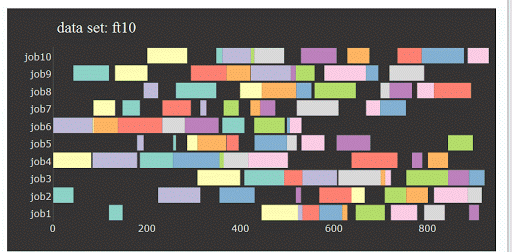A standard problem in continuous time job-scheduling is to forbid jobs to overlap:

 \begin{align}&S_i \ge E_j\\ &\text{or}\\ &S_j \ge E_i\end{align}

where $$S_i$$ and $$E_i$$ are start and finishing times of job $$i$$:In a MIP this is usually formulated as :

 \begin{align}&S_i\ge E_j - M\delta_{i,j}\\ &S_j \ge E_i - M(1-\delta_{i,j})\\ &\delta_{i,j} \in \{0,1\}\end{align}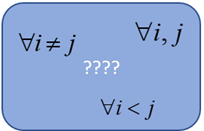##### Best choice: $$\forall i<j$$

If we compare job $$i$$ with $$j$$, we no longer need to check job $$j$$ and $$i$$. So often we implement the constraints only for the combinations $$i<j$$ (of course $$\forall j>i$$ would be just as valid).

In this post I want to investigate a bit what happens if we choose a different set of combinations over which we apply the constraints.

##### Case 2: $$\forall i,j$$

What happens if we are sloppy with this and compare for all $$i,j$$? If $$i=j$$, we introduce an infeasibility that is easily detected by the solver. Some solvers do report:

Model is infeasible or unbounded

which is a little bit less helpful. In any case, the model will not solve if the modeler applies these constraints for all $$i,j$$.

##### Case 3: $$\forall i\ne j$$

Now, what happens if we use $$\forall i\ne j$$ instead of $$\forall i<j$$? This is actually something I see quite often in models (see  for an example). The solver (or modeling system) will not provide any feedback on this. It will solve the model and provides correct solutions in both cases.

What about solution times? There are two things to consider:

1. The number of binary variables $$\delta_{i,j}$$ increases two-fold. However, implicitly the model enforces $$\delta_{i,j}=1-\delta_{j,i}$$. If a variable $$\delta_{i,j}$$ is fixed to zero or one, automatically $$\delta_{j,i}$$ will also assume an integer value. The total impact of this on the node count of MIP solver is not clear to me. Indeed, the number of nodes is not uniformly better using either formulations.
2. The number of equations (and variables) involved increases by a factor of 2. This should slow down the LP relaxations solved by the MIP solvers. I would expect the speed in terms of the number of nodes per second or Simplex iterations per second to decrease. This turns out to be an important factor.

Let’s try this out on a smallish but difficult job shop problem .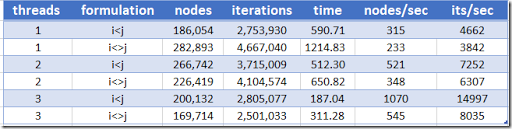Indeed we see no consistency in the node count. However the number of nodes per second and iterations per second behaved as I predicted. The total time is affected by this in such a manner that the funny difference in node count is not as significant as the difference in speed solving the LP relaxations. Even in cases where the node count or iteration count is better for the $$i\ne j$$ model, it still loses out on total time. I.e. $$i<j$$ is a winner, but may be for a different reason than you might think.

Conclusion: please use $$i<j$$ when implementing the no-overlap equations.

##### References
1. Alan Manne, On the job-shop scheduling problem, Operations Research, Vol. 8, No. 2, March-April 1960.
2. ojAlgo Linear Optimization - Preventing work shift overlaps?, https://stackoverflow.com/questions/46823121/ojalgo-linear-optimization-preventing-work-shift-overlaps. I am not familiar with ojAlgo, but the presented model in the answer does seem to implement just $$i \ne j$$.
3. Data set ft10 from H. Fisher, G. L. Thompson, Probabilistic learning combinations of local job-shop scheduling rules, in: J. F. Muth, G. L. Thompson (eds.),  Industrial Scheduling, Prentice Hall, Englewood Cliffs, N.J., 1963.

## Thursday, October 26, 2017

### Profiling in visual studio

Somehow, I am often struggling to understand the output of profilers. This report of Visual Studio actually makes sense to me: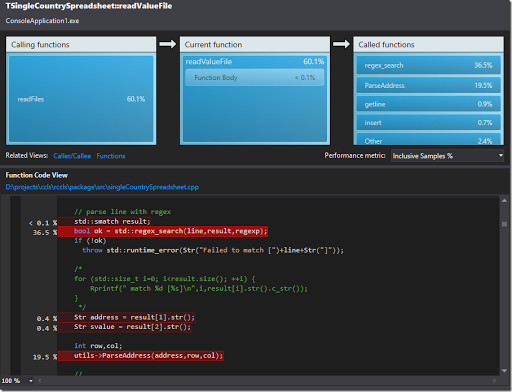I like the code highlighting. It gives a really quick overview of where most of the time is spent. (Most of the time in parsing some files is spent in dealing with the regular expression; I am happy: no obviously poor performance somewhere in my code. Interestingly, also when parsing the Excel formulas I spent most of the time parsing Excel addresses – again using a regular expression.)

## Monday, October 23, 2017

### “Coda is a next-generation spreadsheet designed to make Excel a thing of the past”Picture from 

Not so easy in my opinion. Excel is the workhorse in many workplaces.

## Thursday, October 19, 2017

### Pareto fronts in Z3: a large data set

In  a small multi-objective MIP is solved with Z3. Here we try a larger problem, an engineering design problem. It has three objectives and 2029 non-dominated solutions. The solution in the F space (the objectives) looks like: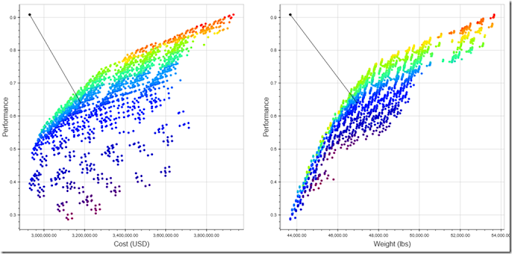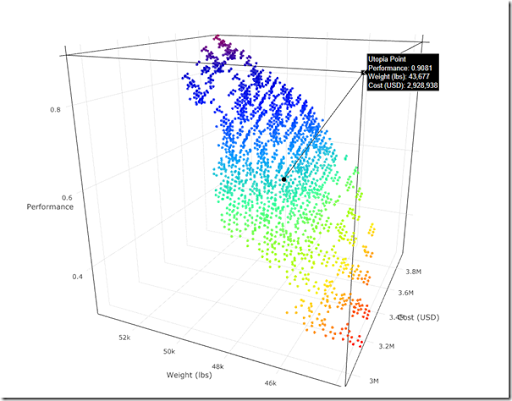To make life easier for Z3, I converted the objectives to integer values. The good news is that Z3 finds the same number of solutions. The bad news: for this problem it is not very fast: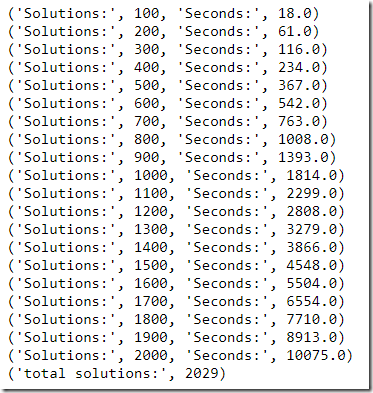We see it is getting slower and slower: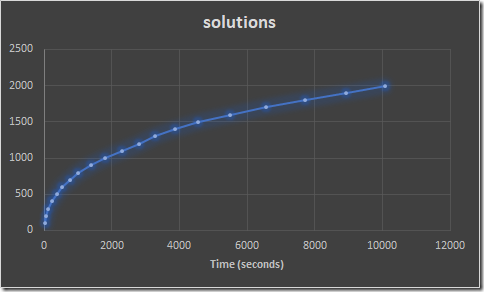The first 500 solutions are found in a rate of about a second per solution. Later on this becomes 10 seconds per solution.

##### Brute force

A brute force method for this problem is:

1. Generate all feasible integer solutions for this problem. I used Cplex for this, using the solution pool technology. I get about 4.8 million solutions.
2. Filter out all dominated solutions. There are 2029 remaining non-dominated solutions.

This method takes about 20 minutes (i.e. 1200 seconds).

##### References
1. http://yetanothermathprogrammingconsultant.blogspot.com/2017/10/multi-objective-mixed-integer.html

## Wednesday, October 11, 2017

### Multi-objective Mixed Integer Programming and Z3Vilfredo Federico Damaso Pareto (1848-1923) [link]

The tool $$\nu Z$$ [1,2] is an optimization extension to the SMT solver Z3 . It can actually generating Pareto optimal solutions for MIP problems, using an algorithm based on . In [4,5] a small example model is presented:

 \bbox[lightcyan,10px,border:3px solid darkblue]{ \begin{align}\max\>&x_1 – 2x_2\\\max\>&-x_1+3x_2\\ &x_1 \le 2x_2\\&x_1,x_2 \in \{0,1,2\}\end{align}}

There are 5 different Pareto optimal solutions. Let’s see how we can implement this in Z3 using Python: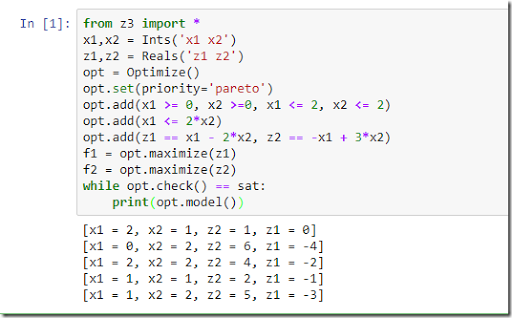This looks promising. I have some large instances lying around. I am curious how this solver will behave on those.

##### A real surprise

We have to be very careful when using floating point coefficients. If we divide the first objective by 2, we should get the same Pareto front. However what we see is: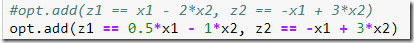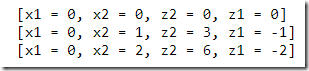What happened here? No, error messages, no warnings, but obviously the results are wrong. We can see what happened by printing: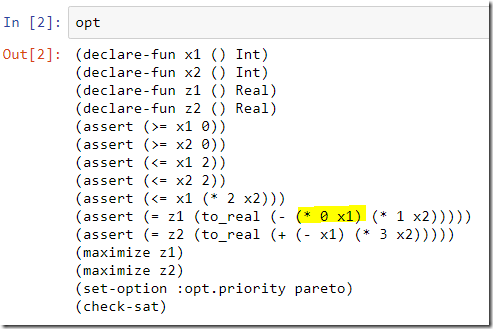Our 0.5 has become 0! (I am yelling now: keep your hands off my data!).

After some experimentation, I came up with the following workaround: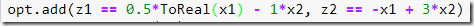Now we get: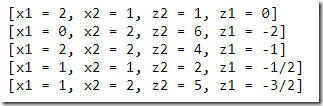The results for z1 indicate the solver actually reports rational values. Indeed the printed model has: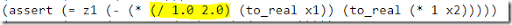(A bit funny. I would expect 0.5 or (/1 2) but not this mixture.)

See  for some background on why this is happening. (I think I would never do this rounding without raising some kind of exception: it is just too dangerous. A little bit ironic that Z3 is used a lot to do program correctness verification.).

##### References
1. Nikolaj Bjørner, Anh-Dung Phan, and Lars Fleckenstein, $$\nu Z$$ - An Optimizing SMT Solver, https://www.microsoft.com/en-us/research/wp-content/uploads/2016/02/nbjorner-nuz.pdf
2. Nikolaj Bjørner and Anh-Dung Phan, $$\nu Z$$ - Maximal Satisfaction with Z3, in Proceedings of International Symposium on Symbolic Computation in Software Science (SCSS), 2014, https://easychair.org/publications/open/xbn
3. The Z3 Theorem Prover, https://github.com/Z3Prover/z3
4. Generating all non-dominated solutions in a multi-objective integer programming model, http://yetanothermathprogrammingconsultant.blogspot.com/2010/02/generating-all-non-dominated-solutions.html
5. Sylva, Crema: A method for finding the set of non-dominated vectors for multiple objective integer linear programs, EJOR 158 (2004), 46-55.
6. D. Rayside, H.-C. Estler, and D. Jackson, The Guided Improvement Algorithm for Exact, General-Purpose, Many-Objective Combinatorial Optimization, Technical Report MIT-CSAIL-TR-2009-033, MIT, 2009, https://dspace.mit.edu/bitstream/handle/1721.1/46322/MIT-CSAIL-TR-2009-033.pdf
7. https://stackoverflow.com/questions/46721639/z3-and-interpretation-of-floating-point-coefficients

## Saturday, October 7, 2017

### Modern Distributed Optimization

Summary
Matt Adereth talks about the Black-box optimization techniques, what’s actually going on inside of these black-boxes and discusses an idea of how they can be used to solve problems today. He deep dives into a few of the most popular ones, such as Distributed Nelder-Mead and Bayesian Optimization, and discusses their trade-offs.
The video of this presentation can be found in . The title is a bit misleading: the talk is really only about two algorithms for unconstrained derivative-free optimization (a small subset of the total optimization field).

For a reference, see the excellent review paper .
##### References
1. Modern Distributed Optimization, https://www.infoq.com/presentations/black-box-optimization
2. Rios, L.M. & Sahinidis, N.V., Derivative-free optimization: a review of algorithms and comparison of software implementations, J Glob Optim (2013) 56: 1247, https://doi.org/10.1007/s10898-012-9951-y

## Friday, October 6, 2017

### von Koch and MiniZinc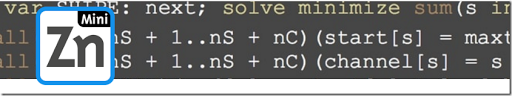A MiniZinc  version of the graceful labeling model in  was sent to me . This looks actually quite nice, so therefore I reproduce it here:

 include "globals.mzn"; %% Definition of data% % Number of nodes in the graphint: nodes;set of int: Nodes = 1..nodes; % Connectionsint: connections;set of int: Connections = 1..connections;array[Connections,1..2] of int: ConnectionEdges; %% The following data could be located in a minizinc data file instead.%nodes = 7;connections = 6;ConnectionEdges =          [|  1,  2,          |  1,  4,          |  1,  7,          |  2,  3,          |  2,  5,          |  5,  6,          |]; %% Model proper% array[Nodes] of var 1..nodes: NodeLabel;array[Connections] of var 1..connections: ConnectionLabel; constraint alldifferent(NodeLabel) :: domain; constraint alldifferent(ConnectionLabel) :: domain; constraint forall(connection in Connections) (    ConnectionLabel[connection] = abs(NodeLabel[ConnectionEdges[connection, 1]] - NodeLabel[ConnectionEdges[connection, 2]]));  solve satisfy; output [  "NodeLabels: " ++ show(NodeLabel) ++   " ConnectionLabels: " ++ show(ConnectionLabel) ++   "\n"  ];

MiniZinc comes with an IDE so that makes it easy to edit and run the model: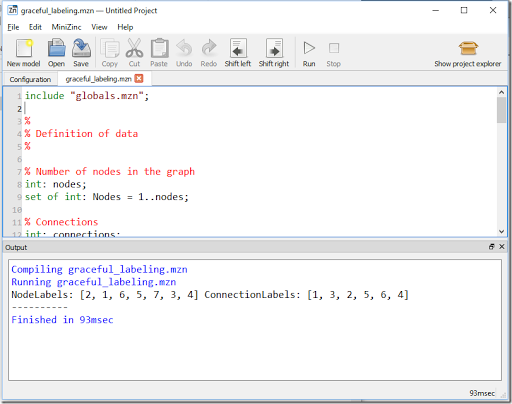This is solved with the Gecode  Constraint Programming solver.

Generating all solutions is just a question of changing a setting:Indeed we get all solutions: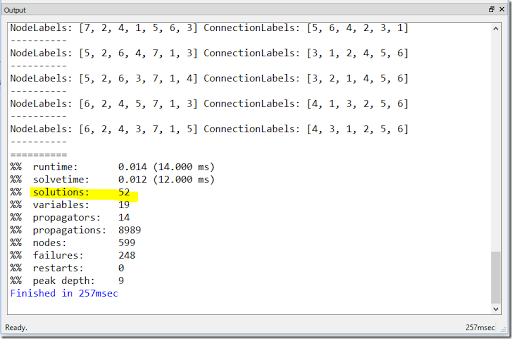##### References
1. http://www.minizinc.org/
2. http://www.gecode.org/
3. von Koch and Z3, http://yetanothermathprogrammingconsultant.blogspot.com/2017/09/von-koch-and-z3.html
4. Mikael Zayenz Lagerkvist, private communication

## Tuesday, October 3, 2017

### Modeling environments for the 21st century?Is this a prediction for the period through December 31, 2100?

Anyway, some interesting points are brought forward. A comparison is made between GAMS, AMPL, AIMMS, solver specific C++ code, Pyomo and JuMP: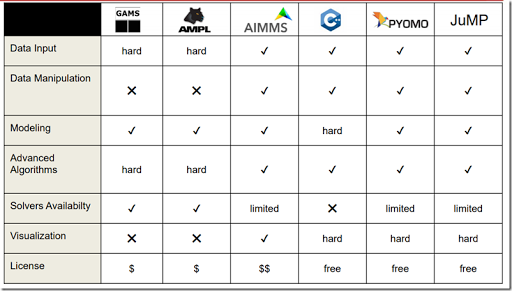Note: my GAMS models are typically >90% data manipulation and <10% model equations (does X means this is not possible or allowed?).

##### References
1. http://egon.cheme.cmu.edu/ewo/docs/EWO_Seminar_03_10_2017.pdf